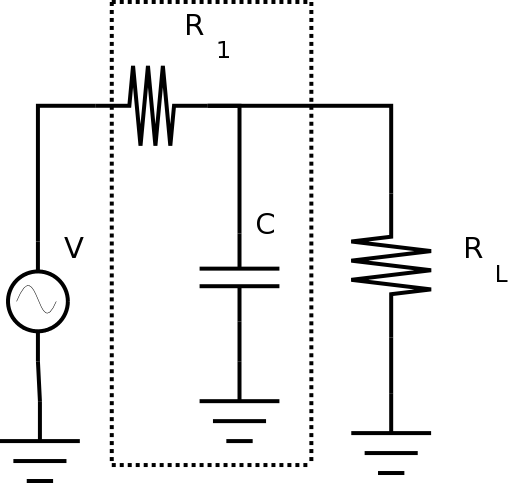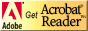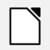# PC221 Analog Electronics I

## RC Circuits

### Objectives

1. To examine capacitor charging and discharging
2. To examine frequency response of an RC circuit
3. To introduce measurement of phase in AC circuits
4. To expand the use of SPICE circuit simulation to include AC analysis.
5. To compare results of analysis, measurement, and simulation.

### Equipment

• digital multimeter, bench power, oscilloscope
• various resistors and capacitors

### Background

One of the common problems in electronics is filtering out unwanted signals from a waveform. One example of the need for this is to remove noise (such as 60Hz noise) from a signal. DC power supplies almost always have some 60Hz ripple (i.e. AC component) due to the AC frequency of our power. What you're going to do in this lab is to use a simple filter to see how effectively it removes noise. To do this you'll use a signal generator to produce a DC signal with ripple, and then try to filter to voltage being provided to a load (in this case, just a fixed resistor.)
To see how the filter works, you'll want to look at the voltages and currents over a range of frequencies.

#### AC circuits with inductors and capacitors

Once capacitors and inductors are included in circuits, analyzing DC and AC parts of the behaviour becomes slightly more complex.
For DC analysis,

• replace all capacitors by an open circuit (i.e. no connection)
• replace all inductors by a short circuit (i.e. a wire)

The AC analysis is more challenging since the impedance of a capacitor or an inductor changes with frequency.
For AC analysis,

• replace all capacitors by the proper impedance of ZC = j / (ω C)
• replace all inductors by the proper impedance of ZL = j ω L

The math is the same, but it now involves complex quantities. The real part of any voltage or current is what is actually measured.

### Procedure

#### Setup

1. Assemble the parts for the circuit shown, using the components as follows: V is a 1V AC sine wave source (of varying frequency) with a DC offset of 5V, like this:The other values are;
• R1 = 100 Ω
• RL = 1.0 kΩ
• C = 1 μF

#### Circuit Measurement: Digital Multimeter

1. Measure the component values with a digital multimeter, and fill them in Table 1.

Here's a link on measuring capacitance.

Quantity Table 1: Component Values and Voltages R1 RL C V (amplitude) V (offset)

#### Circuit Measurement: Oscilloscope

1. Connect the function generator to the oscilloscope. Make sure the scope channel is in DC mode for this. Adjust the wave generator to produce a 1V 100Hz sine wave, with a DC offset of 5V.
When using a wave generator, always check the output with a scope before connecting it to a circuit. Then when you connect it to the circuit, if it changes drastically, there is probably something wrong so you can disconnect it and examine the circuit.

2. Measure DC and AC supply voltage(s) with an oscilloscope, (amplitude and offset), and fill them in Table 1.

#### Circuit Measurement: Choice of Instrument

1. Once the function generator is set up correctly, measure the DC and AC components of the voltage across RL at frequencies of 100Hz, 300Hz, 1kHz, 3 kHz and so on up to 100 kHz using whatever instrument you feel appropriate.

This is one time when the use of AC coupling on the oscilloscope may be useful. When you have a signal with an AC component that is much smaller than the DC component, switching to AC coupling will allow you to expand the scale for the AC component. Switching back to DC coupling will make it possible to change the scale to measure the DC component.

2. Sketch the waveform at 100Hz, 1kHz and 10kHz.

Show your sketches and your results to the lab instructor before continuing.

3. Plot the AC amplitude gain in decibels versus the log of the frequency. This is called a Bode plot.

#### Circuit Visualization: Frequency Sweeps

Many function generators can perform a sweep of frequencies for observing the response of circuits like this. While a varying frequency isn't helpful for taking measurements, it allows you to observe circuit response quickly.Three controls are used for a sweep:

• frequency
• sweep/time
• sweep/rate

When the frequency control is pulled out, the frequency sweeps, rather than staying constant.
The sweep/time control determines the speed of the sweep, and whether it is pulled out determines whether the sweep is linear or logarithmic. (The logarithmic sweep is more useful when the range is very large.)
The sweep/rate control determines the range of frequencies to sweep; it goes from zero (i.e. DC) to some percentage of the frequency chosen by the frequency dial. (Max allows it to sweep all the way up to the set frequency.)

1. Set the dial for a frequency of 100 kHz, and adjust the settings to make a sweep over this range.

2. With the oscilloscope connected to show the voltage across RL, run the sweep and observe the result.

Is this a useful feature? Would it be worth using before taking measurements?

Show your sweep to the lab instructor before continuing.

#### Circuit Simulation: Introducing AC analysis

1. SPICE can do many different types of circuit analysis.

Use LTspice or CircuitLab to perform an analysis to observe the DC behaviour of the circuit.
(Hint: You've previously used an analysis that gives the DC behavior of a circuit.)

Be sure to use the component values and supply voltage(s) from Table 1.

2. Perform whatever analysis you need to create traces to compare to the sketches you produced above. (Hint: You've previously used an analysis that shows what waveforms look like over time.)

3. If using LTspice:
Use the information in the LTspice tutorial to do an AC analysis.

If using CircuitLab:
Do a frequency domain analysis.

4. This analysis shows the ac amplitude over a range of frequencies. Note that this is different from a transient analysis.

Your trace will show both amplitude and phase. Figure out how to determine which is which, and how to show each one separately.

In order to make it easy to compare your trace to your Bode plot from measurements, change the vertical scale from "dB" to "logarithmic".

#### Analysis: Kirchhoff's Laws

You can complete the rest of this after you have left the lab. If your results so far are consistent, doing the analysis should be straightforward.
1. Redraw the circuit in both ways (for DC and AC analysis).

2. Analyze the circuits in the two sketches to determine the voltage across RL in each case.

Be sure to use the component values and supply voltage(s) from Table 1.

(Note: You don't need to determine the voltages across the other passive components.)
Remember that for the AC analysis, the resulting voltages and currents will be AC quantities.

To see how the filter works, you'll want to look at the AC and DC components across RL over a range of frequencies.

#### Comparing Results

1. Compare the values from the actual circuit to the values from your analysis and measurements above. Particularly, compare your Bode plot to your AC analysis.

Before you leave the lab, have the lab instructor sign your lab notebook immediately after your last entry.

## ResourcesIf you need to update a browser, you might try Firefox which is
• free
• open source
• available for several platformsSince this page uses cascading style sheets for its layout, it will look best with a browser which supports the specifications as fully as possible.

If you are looking for an office package, with a word processor, spreadsheet, etc., you might try LibreOffice which is
• free
• open source
• available for several platformsGo to the main page for the Department of Physics and Computer Science.

Wilfrid Laurier University